Question
program should be in c language. thank you for helping me.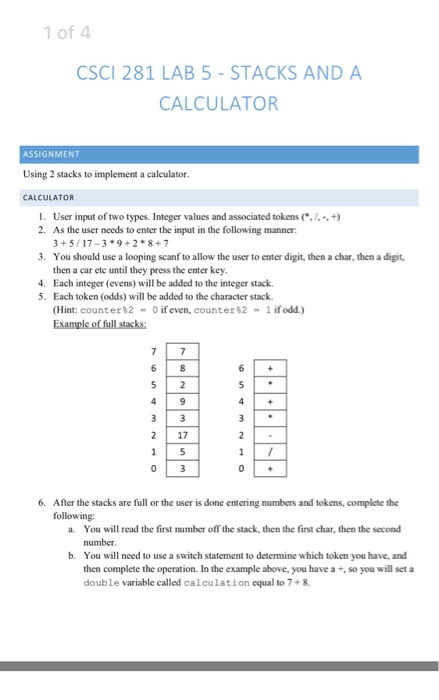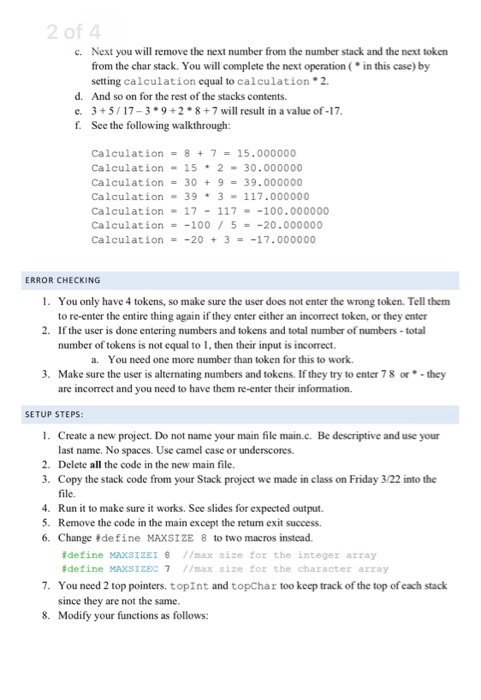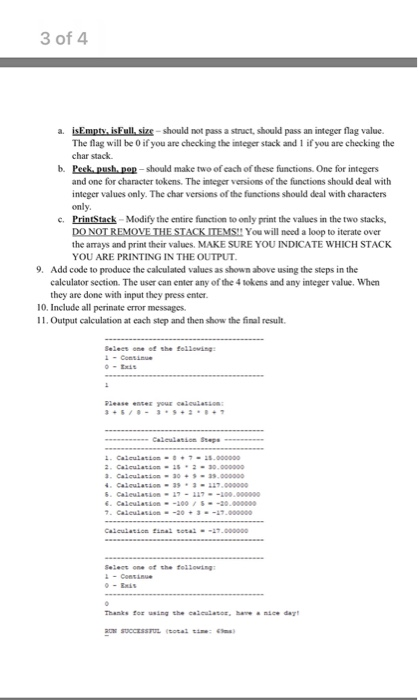#include <stdio.h>
#include <stdlib.h>

#define MAXSIZEI 8
#define MAXSIZEC 7

int *p;
char *c;
int *topInt; /* points to top of stack of int */
int *topChar; /* points to top of stack of char */
int *bottomInt;
char *bottomChar;

void pushInt(int i)
{
if(p > bottomInt) {
printf("Stack Full\n");
return;
}
*p = i;
p++;

}
void pushChar(char i)
{
if(c > bottomChar) {
printf("Stack Full\n");
return;
}
*c = i;
c++;
}

int popInt(void)
{
p--;
if(p < topInt) {
printf("Stack Underflow\n");
return 0;
}
return *p;
}
char popChar(void)
{
c--;
if(c < topChar) {
printf("Stack Underflow\n");
return 0;
}
return *c;
}

int main(void)
{
int a, b;
char s;
char value;
int digit1;
int digit2;
char token;
double calculation = 0.0;

p = (int *) malloc(MAXSIZEI*sizeof(int)); /* get stack memory */
if(!p) {
printf("Allocation Failure\n");
exit(1);
}
topInt = p;
bottomInt = p + MAXSIZEI-1;

c = (char *) malloc(MAXSIZEC*sizeof(char)); /* get stack memory */
if(!c) {
printf("Allocation Failure\n");
exit(1);
}
topChar = c;
bottomChar = c + MAXSIZEC-1;

int i = 1;

while(i=10){

printf("********************************************\n");
printf("Select one of the following \n");
printf("1 Continue \n");
printf("0 Exit \n");
printf("********************************************\n");
scanf("%d", &digit1);
if(digit1 == 0)
exit(0);

do {
//printf("Enter digit1\n");
scanf("%d", &digit1);
pushInt(digit1);
//printf("Enter token\n");
scanf("%c", &token);
//scanf("%c", &token);
pushChar(token);
//printf("Enter digit2\n");
scanf("%d", &digit2);
pushInt(digit2);

scanf("%c", &value);
} while(value != '\n');
calculation = 0.0;
while(p>topInt){
a = popInt();
token = popChar();
b = popInt();

switch(token) {
case '+':
calculation = calculation +(a+b);
break;
case '-':
calculation = calculation +(b-a);
break;
case '*':
calculation = calculation +(b*a);
break;
case '/':

if(a==0) {
printf("Divide by 0.\n");
break;
}
calculation = calculation +(b/a);
break;
}
}
printf("********************************************\n");
printf("Calculation final total %f\n", calculation);
printf("********************************************\n");

}
return 0;
}

#### Earn Coins

Coins can be redeemed for fabulous gifts.

Similar Homework Help Questions
• ### Java language: C Write a game program that allows the user to collect 4 tokens hidden...

Java language: C Write a game program that allows the user to collect 4 tokens hidden in an array (cells) of size 10. The program asks the user to select a cell. Determine if there is a token in that cell or not, and give the user appropriate feedback. This program should keep letting user to select until the all tokens are collected. The program should display the total number of selections. Use rand_position Example input/output: There are four tokens....

• ### Infix Expression Evaluator For this project, write a C program that will evaluate an infix expression. The algorithm REQ...

Infix Expression Evaluator For this project, write a C program that will evaluate an infix expression. The algorithm REQUIRED for this program will use two stacks, an operator stack and a value stack. Both stacks MUST be implemented using a linked list. For this program, you are to write functions for the linked list stacks with the following names: int isEmpty (stack); void push (stack, data); data top (stack); void pop (stack); // return TRUE if the stack has no...

• ### Infix Expression Evaluator For this project, write a C program that will evaluate an infix expression. The algorithm REQ...

Infix Expression Evaluator For this project, write a C program that will evaluate an infix expression. The algorithm REQUIRED for this program will use two stacks, an operator stack and a value stack. Both stacks MUST be implemented using a linked list. For this program, you are to write functions for the linked list stacks with the following names: int isEmpty (stack); void push (stack, data); data top (stack); void pop (stack); // return TRUE if the stack has no...

• ### this assignment should be delivered in C language Program 4 will prompt the user for one...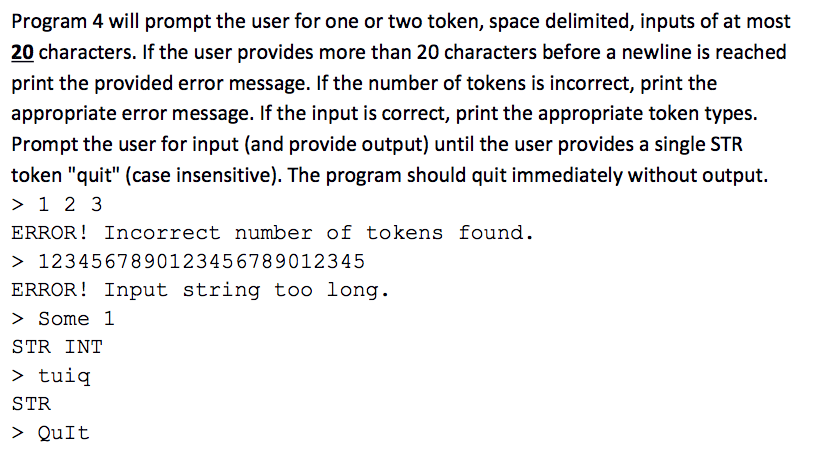this assignment should be delivered in C language Program 4 will prompt the user for one or two token, space delimited, inputs of at most 20 characters. If the user provides more than 20 characters before a newline is reached print the provided error message. If the number of tokens is incorrect, print the appropriate error message. If the input is correct, print the appropriate token types. Prompt the user for input (and provide output) until the user provides a...

• ### Hello, please correct the following C++ CODE it is not working on visual studio: (I WILL RATE, NO...

Hello, please correct the following C++ CODE it is not working on visual studio: (I WILL RATE, NO INCOMPLETE OR WRONG SOLUTIONS PLEASE) // CPP program to evaluate a given // expression where tokens are // separated by space. #include using namespace std; // Function to find precedence of // operators. int precedence(char op){ if(op == '+'||op == '-') return 1; if(op == '*'||op == '/') return 2; return 0; } // Function to perform arithmetic operations. int applyOp(int a,...

• ### Here is the code I have so far. I'm trying to figure out how to implement...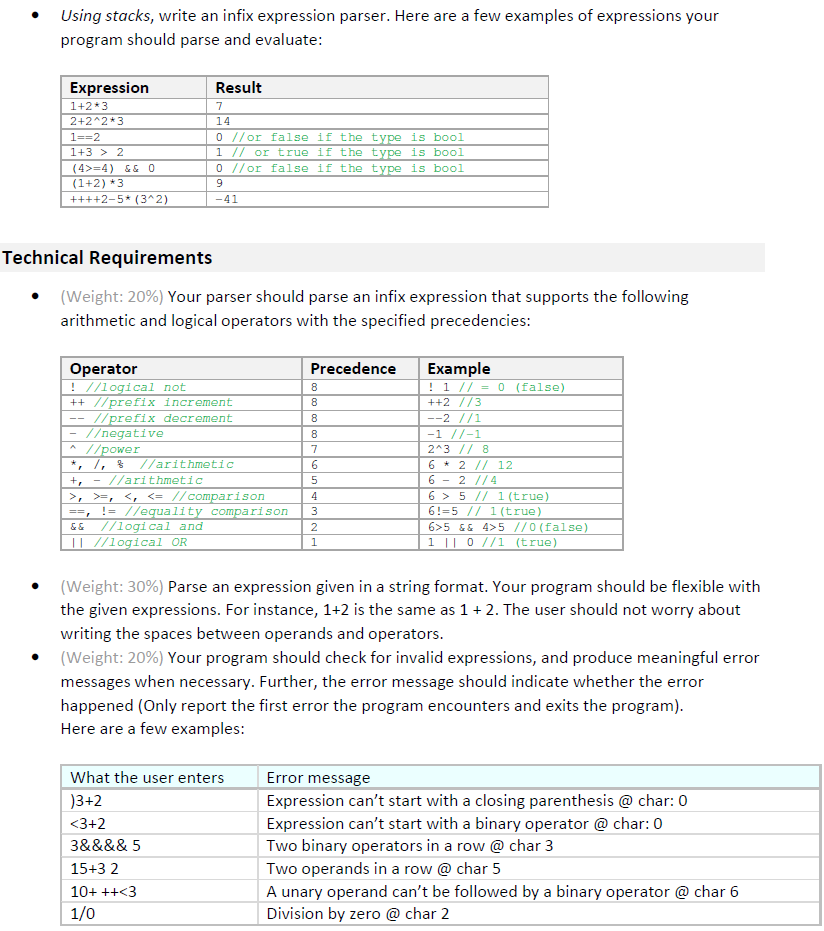Here is the code I have so far. I'm trying to figure out how to implement a boolean and use precedence. The 2nd expression should be 14 but it comes out as 28 so I'm definitely not understanding. #include <stack> #include <iostream> #include <string> using namespace std; // Function to find precedence of // operators. int precedence(char op) {    if (op == '+' || op == '-')        return 1;    if (op == '*' || op ==...

• ### You are to write a program that implements a Reverse Polish Notation Calculator in C using...

You are to write a program that implements a Reverse Polish Notation Calculator in C using BISON and FLEX, You only have to edit the BISON and FLEX files. Link to the files to start and have a general view of the program: https://www.dropbox.com/sh/83yzs66jhftqj5b/AABZcY9Qwl84JdUFnYpQaZk9a?dl=0 Reverse Polish Notation is a mathematical notation in which every operator follows all of its operands. It is sometimes called postfix notation, and does not require any parentheses as long as each operator has a fixed...

• ### Write a C# program (Integer Math Calculator, the programming requirements are as follows: When typing in...

Write a C# program (Integer Math Calculator, the programming requirements are as follows: When typing in 3+4, 10-5, 12*12, 15/5 from console, the program should give the right answers on screen like 7, 5, 144, 3. This is what I have so far: namespace ConsoleApplication3 { class Program { static void Main(string[] args) { String input; do { Console.Write("Type int values to calulate or stop to exit: "); input = Console.ReadLine(); if (input.ToLower() != "stop") { char[] delimiters = {...

• ### HELP NEEDED in C++ (preferred) or any other language Write a simple program where you create...

HELP NEEDED in C++ (preferred) or any other language Write a simple program where you create an array of single byte characters. Make the array 100 bytes long. In C this would be an array of char. Use pointers and casting to put INTEGER (4 byte) and CHARACTER (1 byte) data into the array and pull it out. Make sure you can access both character and integer data at any location in the array. Read data from a text file....

• ### Total point: 15 Introduction: For this assignment you have to write a c program that will...

Total point: 15 Introduction: For this assignment you have to write a c program that will take an infix expression as input and display the postfix expression of the input. After converting the postfix expression, the program should evaluate the expression from the postfix and display the result. What should you submit? Write all the code in a single file and upload the .c file. Problem We as humans write math expression in infix notation, e.g. 5 + 2 (the...